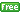www.gllamm.org

## How do I fit IRT models for binary responses?

 Title Fitting binary IRT models in gllamm Author Minjeong Jeon, University of California, Berkeley Date July 2012

Item response data are for test or questionnaire data with responses yij to I items i by J persons j. It is assumed that a continuous latent trait θj, such as ability in the case of test items, explains the item responses via a model such as

logit[P(yij=1|θj)] = θj - βi

where βi is the item difficulty. The model has one parameter per item and is called a one-parameter logistic item response model. A discrimination parameter λi is sometimes introduced to allow the effect of the latent trait on the log-odds of a correct response to differ between items,

logit[P(yij=1|θj)] = λiθj - βi

### Data preparation

The data must be in long form, with all yij for persons j and items i in one variable. The data also require person and item identifiers and item indicator (or dummy) variables. For instance, if there are two students in school 1 who answered two items. Your data should look like this:

```pid   item  y   i1  i2
1       1   0   1   0
1       2   1   0   1
1       1   1   1   0
2       2   0   0   1
...
```
`pid` is the person identifier and `item` is the item identifier. `y` represents item responses and `i1` and `i2` are two item indicator (or dummy) variables.

### One-parameter IRT models in gllamm

Suppose there are 5 binary items in the data. The syntax for fitting one-parameter IRT model is

```gllamm y i1-i5, i(pid) family(bin) link(logit) noconstant  adapt
```
`i()` specifies the person identifier.

The `family()` and `link()` options specify the conditional distribution of the responses and the link function. I used the `logit` link for the example binary data, to specify a one-parameter logistic item response model. One can choose `logit`, `probit`, or `cll` (complementary log-log) links for binary responses.

The `noconstant` option means that we omit the constant in the model so that all the 5 item dummy variables can be used as predictors.

The `adapt` option means that we use the adaptive quadrature method.

### Two-parameter IRT models in gllamm

To fit two-parameter IRT models, we need to specify equations for the discrimination parameters, which are the factor loadings for latent variables (or person random effects or abilities). The syntax for fitting two-parameter IRT model is

```eq load: i1-i5
```
Note that in the first line we define an equation, named `load` for items 1 to 5 using the `eq` command. And then the `eqs()` option is used to specify the variables `i1` to `i5` in the linear combination of variables that multiplies the latent variable in the model.

To speed up estimation, we may reduce the number of quadrature points in the `nip()` option. The default number of points is `nip(8)`. Keep in mind that by reducing the number of quadrature points, you may lose precision of estimates to some degree.

Lastly, note that in this model formulation, the discrimination parameter for the first item is constrained to 1 for model identification.

### Three-parameter IRT models in gllamm

There is no standard way of fitting a three-parameter IRT model in `gllamm`, but it is possible to fit the model if the guessing parameters are known or via a profile likelihood approach. (See Rabe-Hesketh, S. and Skrondal, A. (2007). Multilevel and latent variable modelling with composite links and exploded likelihoods. Psychometrika 72, 123-140. Local )

### Examples and documentation

• Standard one and two-parameter IRT models
• Section 4.1 on One parameter and two parameter item-response models in Rabe-Hesketh, S., Skrondal, A. and Pickles, A. (2004). GLLAMM Manual.U.C. Berkeley Division of Biostatistics Working Paper Series. Working Paper 160.

• Item response models with item and person predictors
• De Boeck, P. and Wilson, M. (Eds.) (2004). Explanatory Item Response Models: A Generalized Linear and Nonlinear Approach. New York: Springer.
• Exercise 10.4 on Verbal aggression data in the book Rabe-Hesketh, S. and Skrondal, A. (2012). Multilevel and Longitudinal Modeling Using Stata (Third Edition). Volume II: Categorical Responses, Counts, and Survival. College Station, TX: Stata Press.
• Skrondal, A. and Rabe-Hesketh, S. (2004). Generalized Latent Variable Modeling: Multilevel, Longitudinal and Structural Equation Models. Chapman & Hall/CRC.

### References

• Embretson, S. E. and Reise, S. P. (2000). Item Response Theory for Psychologists. Mahwah, NJ: Lawrence Erlbaum Associates.
• Rabe-Hesketh, S. and Skrondal, A. (forthcoming). GLLAMM software. In van der Linden, W. J. and Hambleton, R. K. Handbook of Item Response Theory: Models, Statistical Tools, and Applications. Boca Raton, FL: Chapman & Hall/CRC Press, volume 3, chapter 30.
• Rabe-Hesketh, S. and Skrondal, A. (2008). Classical latent variable models for medical research. Statistical Methods in Medical Research 17, 5-32. Local
• Zheng, X. and Rabe-Hesketh, S. (2007). Estimating parameters of dichotomous and ordinal item response models using gllamm.The Stata Journal 7, 313-333.